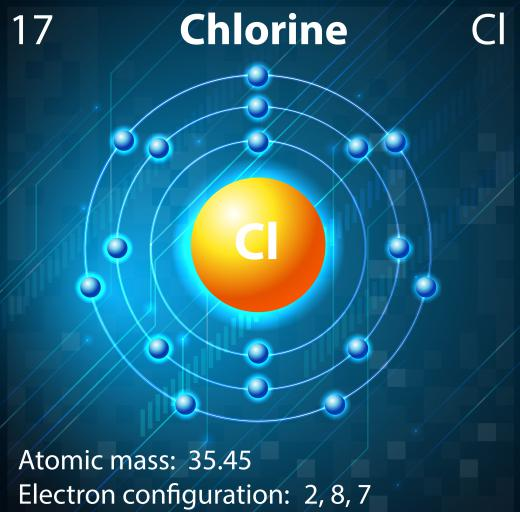Science
Fact Checked

# What Is a Mass Fraction?

Richard Nelson
Richard Nelson

The mass fraction is the ratio of mass that one part of a molecule, mixture, or reaction contributes to the total mass of all its parts. Molecules are groups of atoms, mixtures are groups of molecules, and reactions rearrange atoms into new molecules. All atoms and molecules have a specific mass, and when they are combined, it is often useful to know the mass fraction of a giving atom or molecule in a mixture or reaction. There are several ways to find the mass fraction, but most need a periodic table of elements and basic math skills.

All examples of mass fractions use a fraction calculation. With fractions, it is important to think of parts of the whole, and the mass fraction of any component is the part of the whole mass that a particular component makes up. This leads to dividing the mass of a single component by the total mass in the molecule or mixture to calculate that component’s fraction, denoted Xf. In water there are three atoms: two hydrogen atoms and one oxygen atom. Using the periodic table of elements to find the atomic number of hydrogen and oxygen, each mass fraction can be calculated, and all fractions added together should always equal 1.

Molecules can be combined in a mixture like acid and water. This leads to a similar calculation that gives the mass fraction of each molecule instead of each atom. Water is a common solvent for acid, the mass of which is 18 atomic mass units (amu). Hydrogen chloride is about 36 amu and is often added to water to make hydrochloric acid. If one of each molecule is added to a mixture, or a one-to-one mixture is made, the fraction for each is not ½ because hydrogen chloride weighs twice as much as water per molecule.In hydrogen chloride, there are three hydrogen atoms to every oxygen and chlorine atom.

The same example can be used to show the atomic fraction instead of the molecular fraction. To calculate the amount of mass certain atoms contribute to the total mass, it is first necessary to add up all the same atoms and make a chart. In hydrogen chloride, there are three hydrogen atoms to every oxygen and chlorine atom. When hydrogen’s mass fraction is calculated, it becomes three times the atomic number divided by the total mass, which is about 54 amu. In this example, hydrogen contributes 0.056 of the total mass because three times 1 amu divided by 54 amu is equal to 0.056.

## You might also Like